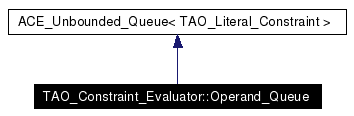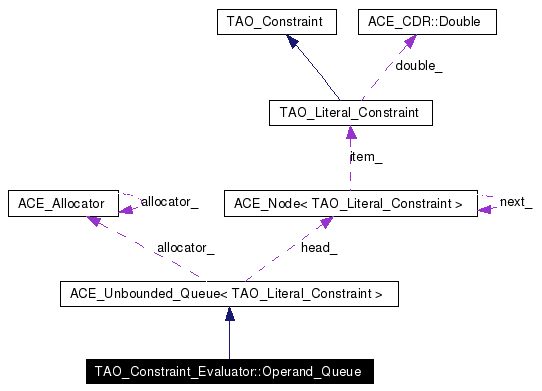# TAO_Constraint_Evaluator::Operand_Queue Class Reference

Inheritance diagram for TAO_Constraint_Evaluator::Operand_Queue:[legend]
Collaboration diagram for TAO_Constraint_Evaluator::Operand_Queue:[legend]
List of all members.

## Public Member Functions

Operand_Queue (void)
TAO_Literal_Constraintget_left_operand (void)
In a binary operation, obtain the left operand.
TAO_Literal_Constraintget_right_operand (void)
In a binary operation, obtain the right operand.
TAO_Literal_Constraintget_operand (void)
In a unary operation, obtain the only operand.
void dequeue_operand (void)
Remove an operand from the queue.

## Constructor & Destructor Documentation

 TAO_Constraint_Evaluator::Operand_Queue::Operand_Queue ( void )

## Member Function Documentation

 void TAO_Constraint_Evaluator::Operand_Queue::dequeue_operand ( void )
 Remove an operand from the queue.

 TAO_Literal_Constraint & TAO_Constraint_Evaluator::Operand_Queue::get_left_operand ( void )
 In a binary operation, obtain the left operand.

 TAO_Literal_Constraint & TAO_Constraint_Evaluator::Operand_Queue::get_operand ( void )
 In a unary operation, obtain the only operand.

 TAO_Literal_Constraint & TAO_Constraint_Evaluator::Operand_Queue::get_right_operand ( void )
 In a binary operation, obtain the right operand.

The documentation for this class was generated from the following files:
Generated on Thu Feb 10 21:03:41 2005 for TAO_CosTrader by1.3.9.1